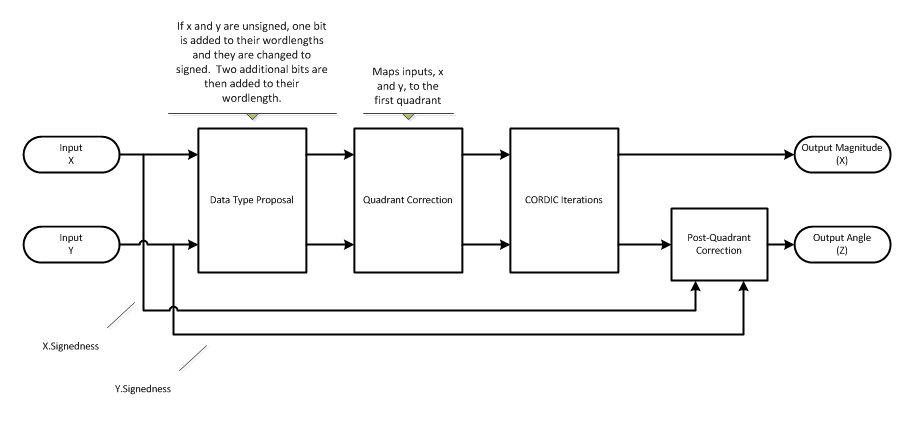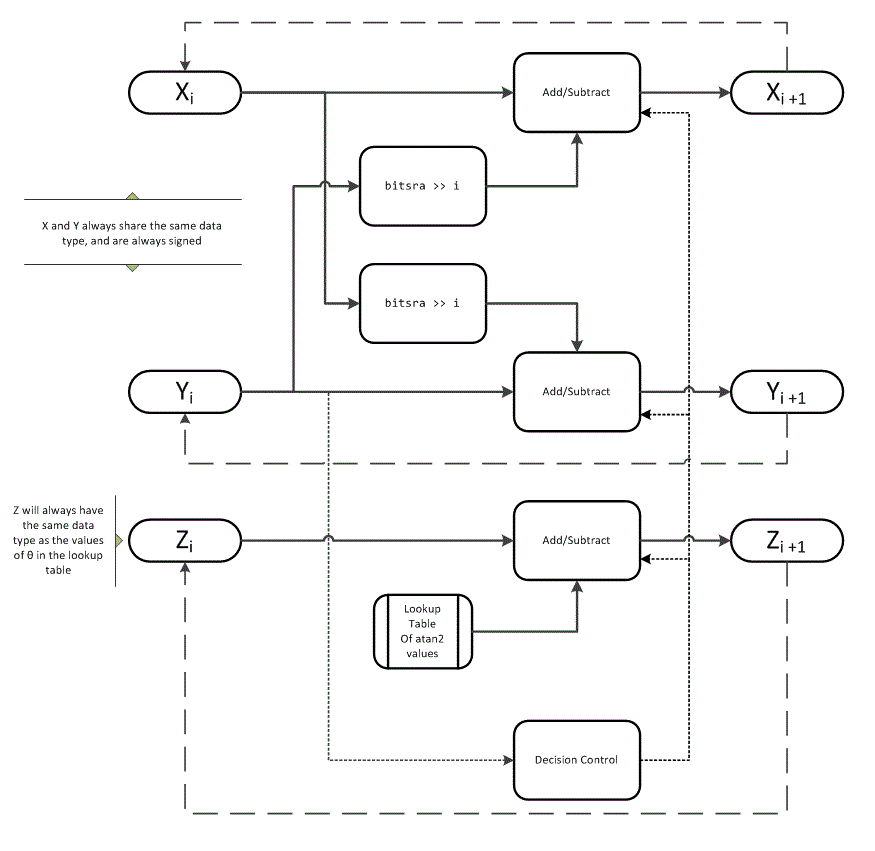# cordicangle

CORDIC-based phase angle

## Syntax

```theta = cordicangle(c) theta = cordicangle(c,niters) ```

## Description

`theta = cordicangle(c)` returns the phase angles, in radians, of matrix `c`, which contains complex elements.

`theta = cordicangle(c,niters)` performs `niters` iterations of the algorithm.

## Input Arguments

 `c` Matrix of complex numbers `niters` `niters` is the number of iterations the CORDIC algorithm performs. This argument is optional. When specified, `niters` must be a positive, integer-valued scalar. If you do not specify `niters`, or if you specify a value that is too large, the algorithm uses a maximum value. For fixed-point operation, the maximum number of iterations is the word length of `r` or one less than the word length of `theta`, whichever is smaller. For floating-point operation, the maximum value is 52 for double or 23 for single. Increasing the number of iterations can produce more accurate results but also increases the expense of the computation and adds latency.

## Output Arguments

 `theta` `theta` contains the polar coordinates angle values, which are in the range [–pi, pi] radians. If `x` and `y` are floating-point, then `theta` has the same data type as `x` and `y`. Otherwise, `theta` is a fixed-point data type with the same word length as `x` and `y` and with a best-precision fraction length for the [-pi, pi] range.

## Examples

Phase angle for double-valued input and for fixed-point-valued input.

```dblRandomVals = complex(rand(5,4), rand(5,4)); theta_dbl_ref = angle(dblRandomVals); theta_dbl_cdc = cordicangle(dblRandomVals) fxpRandomVals = fi(dblRandomVals); theta_fxp_cdc = cordicangle(fxpRandomVals) theta_dbl_cdc = 1.0422 1.0987 1.2536 0.6122 0.5893 0.8874 0.3580 0.2020 0.5840 0.2113 0.8933 0.6355 0.7212 0.2074 0.9820 0.8110 1.3640 0.3288 1.4434 1.1291 theta_fxp_cdc = 1.0422 1.0989 1.2534 0.6123 0.5894 0.8872 0.3579 0.2019 0.5840 0.2112 0.8931 0.6357 0.7212 0.2075 0.9819 0.8110 1.3640 0.3289 1.4434 1.1289 DataTypeMode: Fixed-point: binary point scaling Signedness: Signed WordLength: 16 FractionLength: 13```

collapse all

### CORDIC

CORDIC is an acronym for COordinate Rotation DIgital Computer. The Givens rotation-based CORDIC algorithm is one of the most hardware-efficient algorithms available because it requires only iterative shift-add operations (see References). The CORDIC algorithm eliminates the need for explicit multipliers. Using CORDIC, you can calculate various functions such as sine, cosine, arc sine, arc cosine, arc tangent, and vector magnitude. You can also use this algorithm for divide, square root, hyperbolic, and logarithmic functions.

Increasing the number of CORDIC iterations can produce more accurate results, but doing so increases the expense of the computation and adds latency.

## Algorithms

collapse all

### Signal Flow DiagramsCORDIC Vectoring KernelThe accuracy of the CORDIC kernel depends on the choice of initial values for X, Y, and Z. This algorithm uses the following initial values:

### fimath Propagation Rules

CORDIC functions discard any local `fimath` attached to the input.

The CORDIC functions use their own internal `fimath` when performing calculations:

• `OverflowAction``Wrap`

• `RoundingMethod``Floor`

The output has no attached `fimath`.

## Version History

Introduced in R2011b